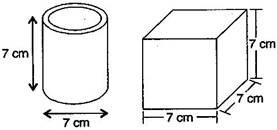# Describe how the two figures below are alike and how they are different. Which box has larger lateral surface areas?Given

Diameter of cylinder = 7 cm

Radius of cylinder (r) = 7 / 2 cm

Height of cylinder (h) = 7 cm

Find out

We have to find out which box has larger lateral surface areas

Solution

The lateral surface area of cylinder = 2πrh

= 2×(22/7)×(7/2)×7 = 154

So, the Lateral surface area of the cylinder is154 cm2

Now, lateral surface area of cube = 4 (side)2=4×72 = 4×49 = 196

The lateral surface area of the cube is 196 cm2

Hence, the cube has a larger lateral surface area.